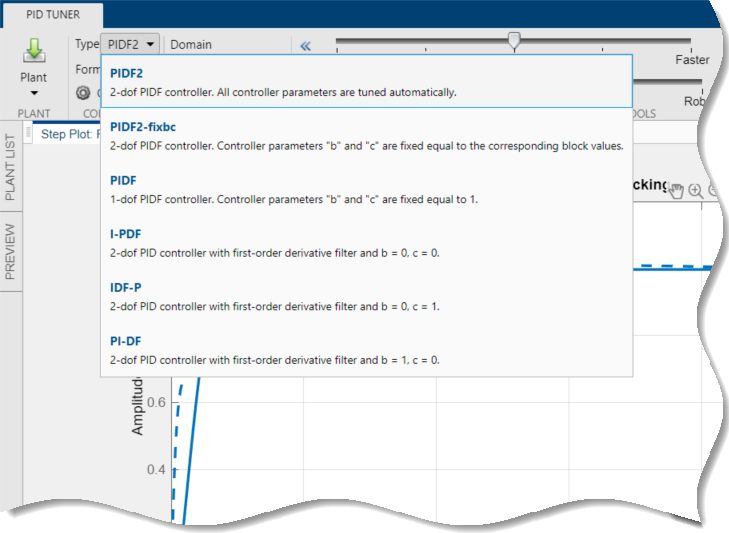Specify PI-D and I-PD Controllers

PI-D and I-PD controllers are used to mitigate the influence of changes in the reference signal on the control signal. These controllers are variants of the 2DOF PID controller.

The general formula of a parallel-form 2DOF PID controller is:

$u=P\left(br-y\right)+I\frac{1}{s}\left(r-y\right)+D\frac{N}{1+N\frac{1}{s}}\left(cr-y\right).$

Here, r and y are the reference input and measured output, respectively. u is the controller output, also called the control signal. P, I, and D specify the proportional, integral, and derivative gains, respectively. N specifies the derivative filter coefficient. b and c specify setpoint weights for the proportional and derivative components, respectively. For a 1DOF PID controller, b and c are equal to 1.

If r is nonsmooth or discontinuous, the derivative and proportional components can contribute large spikes or offsets in u, which can be infeasible. For example, a step input can lead to a large spike in u because of the derivative component. For a motor actuator, such an aggressive control signal could damage the motor.

To mitigate the influence of r on u, set b or c, or both, to 0. Use one of the following setpoint-weight-based forms:

• PI-D (b = 1 and c = 0) — Derivative component does not directly propagate changes in r to u, whereas the proportional component does. However, the derivative component, which has a greater impact, is suppressed. Also referred to as the derivative of output controller.

The general formula for this controller form is:

$u=P\left(r-y\right)+I\frac{1}{s}\left(r-y\right)-D\frac{N}{1+N\frac{1}{s}}y.$

• I-PD (b = 0 and c = 0) — Proportional and derivative components do not directly propagate changes in r to u.

The general formula for this controller form is:

$u=-Py+I\frac{1}{s}\left(r-y\right)-D\frac{N}{1+N\frac{1}{s}}y.$

The following plot shows u for different PID forms for a step reference. The 1DOF PID controller results in a large spike when the reference changes from 0 to 1. The PI-D form results in a smaller jump. In contrast, the I-PD form does not react as much to the change in r.You can tune the P, I, D, and N coefficients of a PI-D or I-PD controller to achieve the desired disturbance rejection and reference tracking.

Specify PI-D and I-PD Controllers Using PID Controller (2DOF) Block

To specify a PI-D or I-PD Controller using the PID Controller (2DOF) or Discrete PID Controller (2DOF) blocks, open the block dialog. In the Controller menu, select PID.

• For a PI-D controller, enter 1 in the Setpoint weight (b) box, and 0 in the Setpoint weight (c) box.

• For an I-PD controller, enter 0 in the Setpoint weight (b) box, and 0 in the Setpoint weight (c) box.

For an example that demonstrates the PI-D and I-PD controller forms, type ex_scd_pid2dof_setpoint_based_controllers. This opens a model that compares the performance of a 1DOF PID, a PI-D, and an I-PD controller.

Automatic Tuning of PI-D and I-PD Controllers

You can use PID Tuner to automatically tune PI-D and I-PD controllers while preserving the fixed b and c values. To do so:

1. In the model, open the block. In the block dialog box, in the Controller menu, select PID.

2. Click . PID Tuner opens.

3. In PID Tuner, in the Type menu, select PI-DF or I-PDF. PID Tuner retunes the controller gains, fixing b = 1 and c = 0 for PI-D, and b = 0 and c = 0 for I-PD.You can now analyze system responses as described in Analyze Design in PID Tuner.